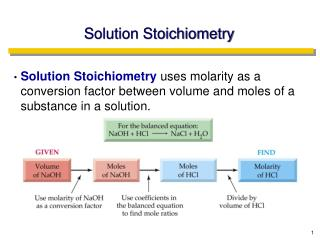DownloadDownload PresentationSolution Stoichiometry

# Solution Stoichiometry

Télécharger la présentation## Solution Stoichiometry

- - - - - - - - - - - - - - - - - - - - - - - - - - - E N D - - - - - - - - - - - - - - - - - - - - - - - - - - -
##### Presentation Transcript

1. Solution Stoichiometry • Solution Stoichiometry uses molarity as a conversion factor between volume and moles of a substance in a solution.

2. Solution Stoichiometry • Titration: • A technique for determining the concentration of a solution.

3. Titrations Titration: the process of analyzing composition by measuring the volume of one solution needed to completely react with another solution. This is a special case of a Limiting Reagent! Usually the reaction of an acid with a base.

4. Titrations Analyte: the solution of unknown concentration but known volume. Titrant: the solution of known concentration. Analyte + Titrant → Products Add titrant until all of the analyte has reacted, then detect the excess of titrant.

5. Titrations Equivalence Point: the point at which exactly the right volume of titrant has been added to complete the reaction. Indicator: substance that changes color when an excess of titrant has been added (phenolphthalein, bromocresol green).

6. Titrations Titration Calculations: • Find the number of moles of titrant added to reach the endpoint. • Determine the moles of analyte that must have been present (use stoichiometric coefficients). • Determine the concentration of analyte that must have been present in the flask (use the volume of analyte). • Calculate the concentration of analyte in the original sample.

7. Titrations Example #1: 14.84 mL of an HCl solution of unknown concentration is titrated with standard NaOH solution. At the equivalence point, 25.0 mL of the 0.675 M NaOH has been added. Calculate the concentration of the HCl solution. NaOH + HCl → NaCl + H2O Titrant = ? Analyte = ?(1.14 M)

8. Titrations Example #2: An antacid tablet containing sodium bicarbonate (NaHCO3) and weighing 4.00 g is dissolved in water. The solution is titrated to the equivalence point with 50.0 mL of 0.200 M HCl. Calculate the mass% of sodium bicarbonate in the tablet. NaHCO3 + HCl → NaCl + H2O + CO2 Titrant = ? Analyte = ?(21.0%)